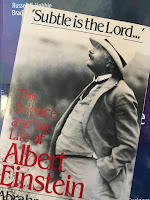Friday, April 29, 2016

The Four Equations of Old Quantum TheorySubtle is the Lord, by Abraham Pais.
In ‘Subtle is the Lord…”: The Science and the Life of Albert Einstein, Abraham Pais illustrates the old quantum theory using four equations:
Does Intermediate Physics for Medicine and Biology introduce students to these four landmark equations? Let us look one by one.

Planck’s law

Planck’s law for blackbody radiation is presented in Sec. 14.8 of IPMB as our Eq. 14.38 (see last week’s post in this blog). Although we don’t delve into the history of this equation, we do analyze it in detail, deriving the Stefan-Boltzmann law and the Wien displacement law (the peak frequency of radiation increases with temperature). Pais writes “It is remarkable that the old quantum theory would originate from the analysis of a problem as complex as blackbody radiation. From 1859 to 1926, this problem remained at the frontier of theoretical physics, first in thermodynamics, then in electromagnetism, then in the old quantum theory, and finally in quantum statistics.”

The Photoelectric Effect

IPMB presents the photoelectric effect equation as Eq. 15.3 in the chapter about the Interaction of Photons and Charged Particles with Matter. However, it is not discussed in the context of light shining on a metal surface. Rather, it describes a photon interacting with tissue. “In the photoelectric effect…the photon is absorbed by the atom and a single electron, called a photoelectron, is ejected. The initial photon energy is equal to…the kinetic energy of the electron…plus the excitation energy of the atom.” The photoelectric effect is the primary mechanism by which low energy photons (soft x-rays, up to photon energies of roughly 100 keV) interact with tissue. It is the main contributor to the tissue cross section at low energies.

The Rydberg Constant

The atomic energy levels of hydrogen, as derived by Niels Bohr, are presented in Eq. 14.8 of IPMB. However, the Rydberg constant is not mentioned in our book except in homework problem 14.4, where the student is asked to “Find an expression for [the Rydberg constant] in terms of fundamental constants.”

The Specific Heat of a Solid

Sorry, but you won’t find Einstein’s equation for the specific heat of a solid in IPMB. In Section 3.1 we do discuss heat capacity. But biology occurs at fairly high temperatures, and human biology is essentially isothermal. The power of Einstein’s equation becomes evident when you examine how the specific heat decreases as the temperature approaches absolute zero. This behavior is critical for understanding low temperature physics, but is irrelevant for physics applied to medicine and biology.